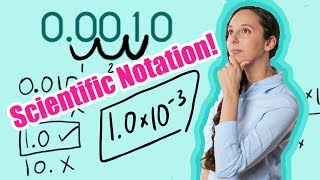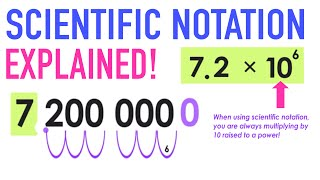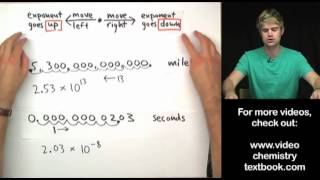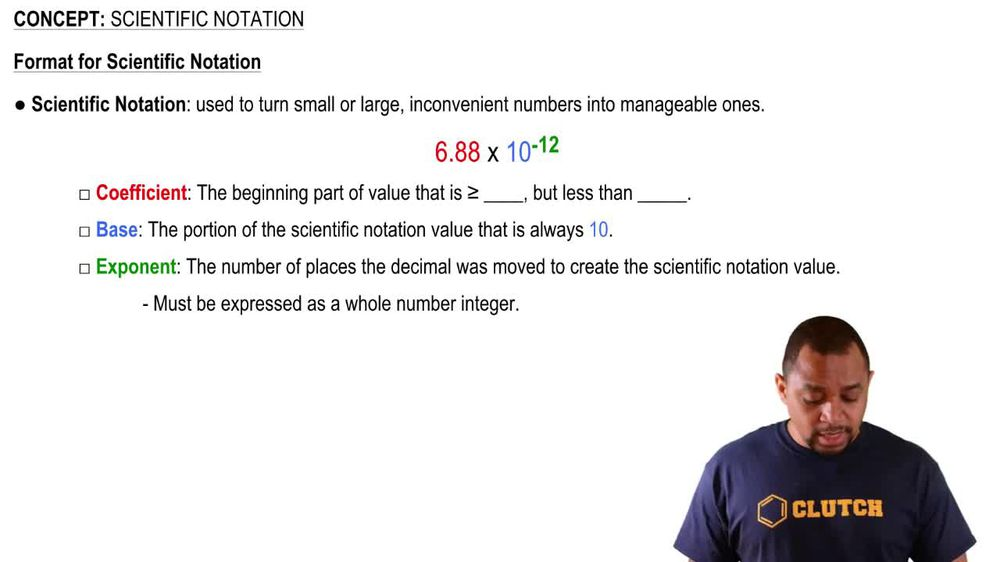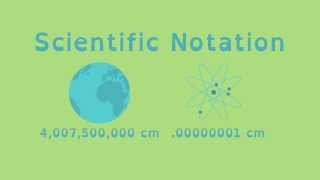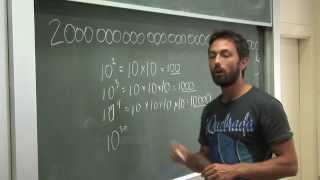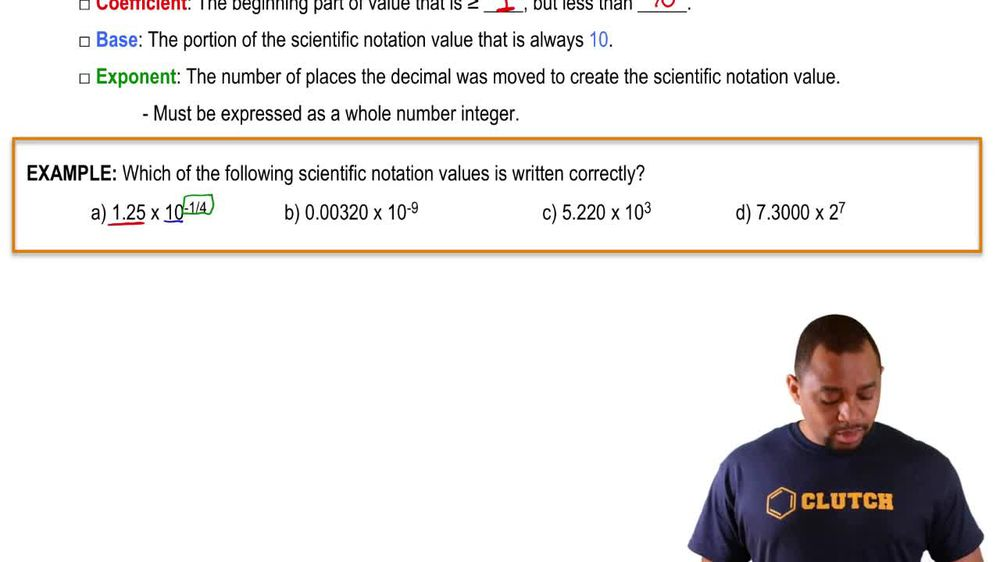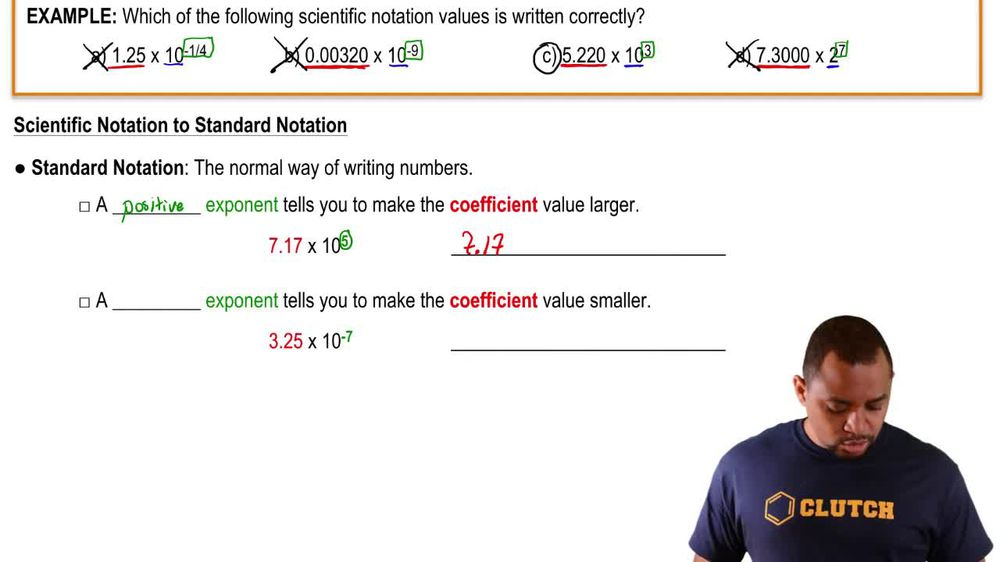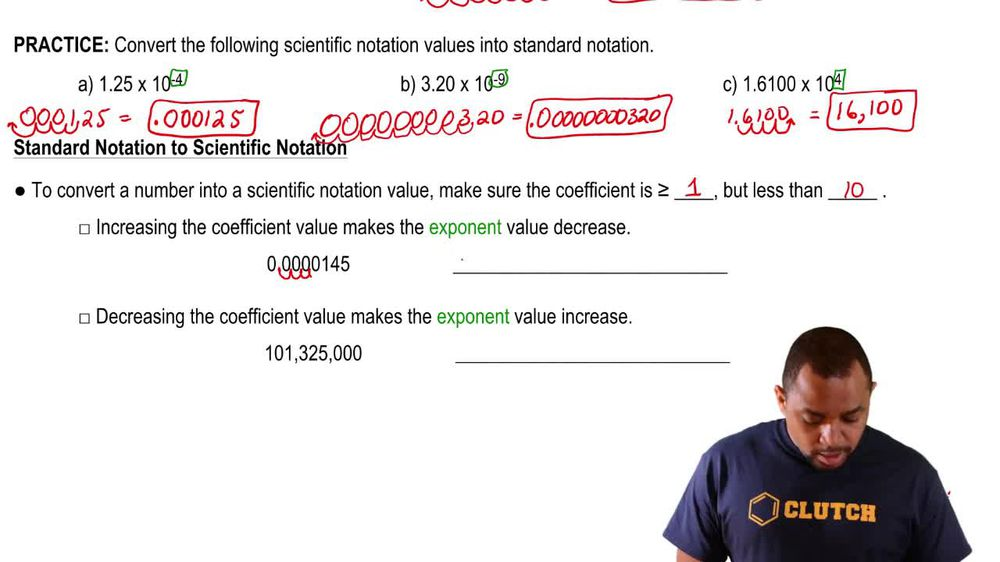Start typing, then use the up and down arrows to select an option from the list.
1. 1. Intro to General Chemistry2. Scientific Notation
Problem

# Convert the following measurements from scientific notation to standard notation. (a) 3.221 * 10-3 mm (b) 8.940 * 105 m (c) 1.350 82 * 10-12 m3 (d) 6.4100 * 102 km

Relevant Solution2m
Play a video:
Hello everyone today. We are being asked to provide the standard notation for the following measurements expressed and scientific notation. So simply put we just have to write it out. So for a for a here We're going to take 2.450. We're gonna take this decimal point. We're going to move it three spaces to the right 123. And this is going to give us 2450. It's important to note that when we have a positive exponent we move to the right. If we have a negative exponents we move to the left for B. We have four 0.54 point 115 times 10 to the negative fourth. Since we have a negative exponent we're gonna take this exponent we're gonna move up four spots to the left 1234. This gives us a new value of zero point followed by three zeros 4115 centimeters for c. We have 3.670 to 1 times 10 to the negative six. We have a negative exponent and therefore we're going to move this decimal spot six paces to the left 123456. This gives us a new value of 0. 0-1 cm cubed and far. Last Question here this is a positive exponent. So we're going to take our 7.2100. And we're gonna move this decimal point behind the (712) spots that give us a new value of 721, test meters. And these are our final answers overall. I hope this helped until next time.